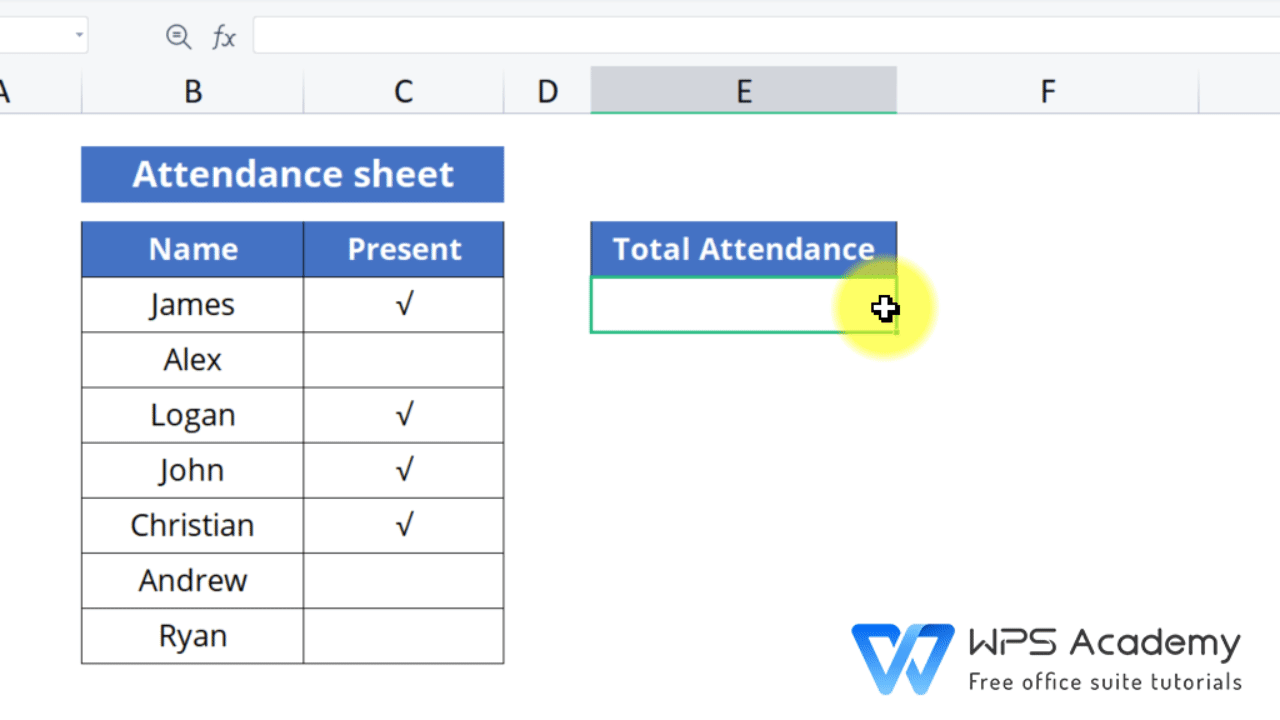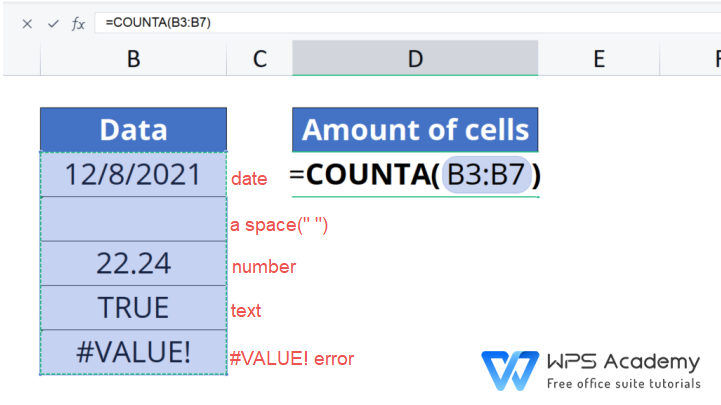# The COUNTA function in excel

November 4, 2021
122 Views

### Graphic skills

In WPS Spreadsheet, the COUNTA function can help us count the amount of cells left non-empty.

· The COUNTA function:

=COUNTA(value1, [value2], ...)

· Use the COUNTA function for total attendance:

In the following example, we use the COUNTA function to calculate total attendance.

1. Enter the formula =COUNTA(C5:C11)

2. Press Enter to get the result.

As we can see, since 4 cells in total are filled with '', the result is '4', with the empty cells skipped.Use the COUNTA function for total attendance

· Other practices with the COUNTA function:

The COUNTA function count the non-empty cells amount, no matter the cell is filled with text, figures, data and time, a space( ), #VALUE! error, etc.

In the following example, Enter the formula =COUNTA(B3:B7). Then, the result will be '5'.If you want to count the cells that fulfill a certain or multiple conditions, please use the COUNTIF function or the COUNTIFS function.

To be office excel advanced, you could learn how to use WPS Office Spreadsheet online in WPS Academy.